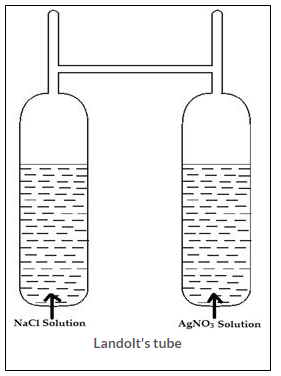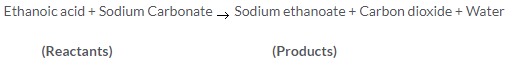## Selina ICSE Solutions for Class 9 Chemistry – Matter and its Composition

Selina ICSE Solutions for Class 9 Chemistry Chapter 1 Matter and its Composition

Exercise 1

Solution 1.

(a) Melting point: The constant temperature at which a solid changes into liquid state by absorbing heat energy is called melting point.

(b) Boiling point: It is the temperature at which a liquid changes into vapour under atmospheric pressure.

(c) Evaporation: The slow passing of molecules of a liquid into gaseous state at a temperature below its boiling point.

(d) Freezing: It is a process in which a liquid changes into solid state by giving out heat energy.

Solution 2.

Boiling point of a liquid can be raised by increasing the atmospheric pressure.

Solution 3.

On heating, solid wax melts into liquid wax, which on further heating, is converted into wax vapours. These changes can be seen in a burning candle. The candle is made up of a solid wax. When we light a candle, the wax near its wick melts. The molten wax rises up the wick and is converted into wax vapour. The wax vapour mixes with oxygen in the air and burns. In a lighted candle, you can see the solid and the liquid states of wax. The vapour of wax can be seen rising from the wick for some time after the candle is put out.Solution 4.

(a) Sublimation: The process by which a solid directly change to its vapour state (or gaseous state) without passing through liquid state and vice versa is called sublimation.

(b) Liquefaction: It is a process of change of state of a substance from gaseous state to liquid state at a particular temperature. It is also known as condensation.

(c) Melting: It is process of changing from solid state to a liquid state at a particular temperature.

(d) Boiling: The process by which a liquid rapidly changes into a gaseous state, by absorbing the heat energy is called boiling.

Solution 5.

(a)

 An atom A molecule Atom is a smallest particle of an element. Molecule is a group of two or more atoms combined together so it is bigger. Atom consists of nucleus (containing protons and neutrons) and electrons. Molecule consists of combination of two or more like or different atoms chemically bound together e.g. H2, HCl, NaCl etc. Atom can neither be seen through naked eye nor through magnifying microscope. Molecule is not visible to naked eye, while can be seen through highly magnifying microscope. Atom cannot be further divided. Molecule can further be divided to give individual atoms. Atoms may or may not have independent existence. Molecules are capable of having independent existence. For example, atom of oxygen (O) has no independent existence while its molecule exists as O2in nature.

(b)

 Boiling Evaporation Boiling is the process in which liquid gets converted into gaseous state. Evaporation is a process in which the liquid gets converted into its gaseous form at any temperature below its boiling point. Boiling occurs at the entire mass of the liquid. That is, it is a bulk phenomenon. Evaporation occurs on the surface of the liquid. That is, it is a surface phenomenon. Boiling occurs rapidly. Evaporation is a slow process. Boiling occurs at a specific temperature. Evaporation occurs at any temperature.

(c)

 Melting Boiling The process of changing from solid state to a liquid state at a particulartemperature is called melting or fusion. The process of change of liquid to vapour form all parts of the liquid at a particular temperature is called boiling. Melting refers to the phenomenon when a solid transforms into a liquid. Boiling refers to the phenomenon when liquid transform into a gas. Example: Melting of ice Example: Boiling of water

(d)

 Gas Vapour A substance exists as a gas at the room temperature and atmospheric pressure. A substance is a solid or liquid under ordinary condition but it is gaseousunder specific conditions. It is present at ordinary conditions of temperature. Its temperature is lower than the boiling point of its liquid state. e.g. – Nitrogen, oxygen. e.g. – Iodine, Camphor

Solution 6.

(a) Water boils of 100oC under 1 atmosphere pressure.
(b) At high altitude water boils below 100oC.
(c) A liquid evaporates below its boiling point.
(d) When a substance is heated kinetic energy of the particles increases.
(e) Solids have the negligible inter-particle space.
(f) Gases have the negligible inter-particle forces.

Solution 7.

(a) Increase in atmospheric pressure
(b) Sulphur
(c) Inter-conversion of state of matter

Solution 8.

(a) Sublimation
(b) Melting
(c) Evaporation
(d) Vaporisation

Solution 9.

(a) Increase in temperature favours Evaporation. When evaporation occurs, remaining liquid becomes cooler. The particles of the liquid absorb heat energy from surroundings to regain energy lost during evaporation which makes the surroundings cold.

(b) Earthen pot has pores which help in evaporation. Some of the water continuously seeps out from these pores. This water absorbs heat of vaporization from the remaining water and gets evaporate. Thus, the remaining water loses heat and gets cooled.

(c) This happens because, when the petrol changes from liquid state to the vapour state, is absorbs heat energy from the palm. The palm thus loses heat and gets cooled.

(d) In humid weather wet clothes take longer time to dry up due to the slow evaporation of water from their surface.

(e) Evaporation is a surface phenomenon. With increase in surface area, evaporation increases. Hot tea in Saucer cools faster than in a cup and hence we can sip faster.

Solution 10.

(a) Naphthalene balls become smaller day by day as they have very weak force of attraction operating between their particles, which break away from other particles from the surface of solid without heating.

(b) In gases the particles are far apart and there is enough space available for compression. Hence, gases can be compressed easily.

(c) Heat energy supplied increases the rate of vibration of the particles and decreases. The inter-particle attraction.

(d) Light has no mass and it does it occupy space. Thus, it is not considered as matter.

(e) According to ‘Law of Conservation of Mass’, “Mass can neither be created nor destroyed in a chemical reaction.” However, it may change from one form to other.

Solution 11.

In summers, we perspire more. Cotton being a good absorber of water helps in absorbing the sweat and exposes it to the atmosphere for evaporation. When sweat evaporates from our body, it takes heat from our body. The heat energy equal to the latent heat of vaporisation is absorbed from the body leaving the body cool.

Solution 12.

Balloon get heat from sun and on heating, the vibration of particles increases and the inter-particle force of attraction between them gets reduced, therefore, balloon bursts.

Solution 13.

Law of conservation of mass: It states that mass can neither be created nor destroyed in a chemical reaction. During any change, physical or chemical, matter is neither created nor destroyed. However it may change from one form to another.

Experimental Verification of Law of Conservation of Mass

Requirements: H-shaped tube called Landolt’s tube, Sodium chloride solution, silver nitrate solution, etc.

Procedure: A specially designed H-shaped tube is taken. Sodium chloride solution is taken in one limb ofthe tube and silver nitrate solution in the other limb as shown in figure. Both the limbs are now sealed and weighed. Now the tubes is averted so that the solutionscan mix up together and react chemically. The reaction takes place and a white precipitate of silver chloride is obtained.The tube is weighed again. The mass of the tube is found to be exactly the same as the mass obtained before inverting the tube. Thus, this experiment clearly verifies the law of conservation of massSolution 14.

Law of conservation of mass is applied to a burning candle. A candle is made of solid wax. When it is lighted, wax near its wick melts and changes into to liquid form. The molten wax rises up the wick and is converted into wax vapours. The wax vapours their mix with oxygen in the air.

Thus, in burning of candle the matter is neither created nor destroyed but one form is changed into the other form.

Solution 15.

The reaction is:Total mass of reactants = (6 g + 5.3 g) = 11.3 g
Total mass of products = (8.2 + 2.2 + 0.9) g = 11.3 g
As the total mass of reactants is equal to the total mass of products. Hence, the reaction follows Law of conservation of mass.

Solution 16.

The reaction will be as follows:
Methane + Oxygen → Carbon dioxide + Water

According to law of conservation of mass,
Total mass of reactants = Total mass of products
Mass of methane + mass of oxygen = Mass of carbon dioxide + Mass of Water
Mass of methane  +  32 g  =  22  +  18 g
Mass of methane = (40 – 32) = 8 g
8g of methane is required.

Solution 17.

Word equation for the reaction is:
Sodium + Chlorine → Sodium Chloride

According to law of conservation of mass,
Total mass of reactants = Total mass of products
Mass of sodium + Mass of chlorine = Mass of sodium chloride
23 g+ Mass of chlorine = 58.5 g
Mass of chlorine = 58.5 – 23 = 35.5 g
35.5 g of chlorine is needed.

Solution 18.

Word equation for the reaction is:
Magnesium + Oxygen → Magnesium oxide

According to law of conservation of mass,
Total mass of reactants = Total mass of products
Mass of magnesium + Mass of oxide = Mass of magnesium oxide
4.8g           +          3.2g     = Mass of magnesium oxide
Mass of magnesium oxide = 8 g

Solution 19.

(a) (iv) No fixed shape and size highly compressible.
(b) (i) The solid starts melting.
(c) (i) evolved
(d) (iv) decreases with increasing pressure.
(e) (iii) Iodine

Solution 20.

(a) does not, melting, boiling
(b) liquid, solid
(c) temperature, temperature
(d) gaseous, sublimation
(e) high and negligible

Solution 21.

(a) freezing
(b) less
(c) sublimation
(d) remains constant

Solution 22.

 Column A Column B (a) Constituent of matter Molecules (b) No compressibility Solid (c) Maximum expansion Gas (d) Conversion of a gas into liquid Condensation